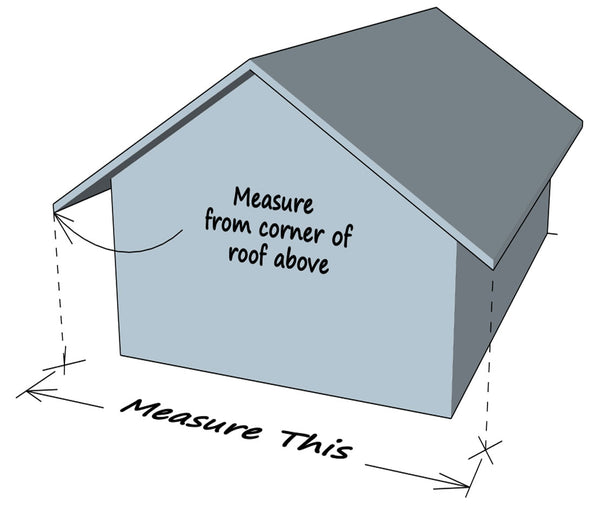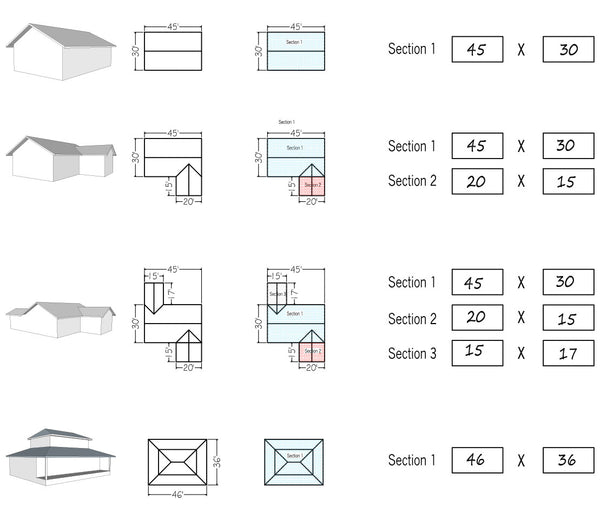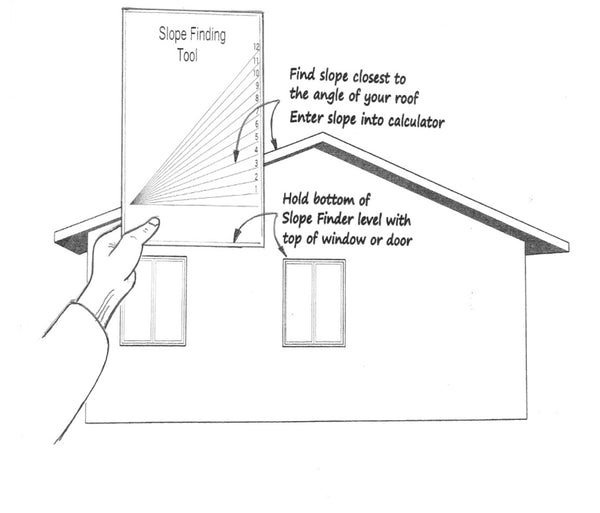FREE SHIPPING
on orders of 5 pails or more
*Click on red cart button on right to apply codes*
• (800) 402-7370
• 6 DAYS A WEEK
• Home
• Measuring the Roof

# HOW TO MEASURE YOUR ROOF

## 4 Simple Steps

STEP 1 - From the ground, measure the distance between the corners of the roof above (see example below). This measures the "foot print" of your roof.STEP 2 - Divide the shape of your roof into rectangular sections (see examples below).Enter the length and width of each section into the calculator.STEP 3 - Print out the Slope Finding Tool below and use it to find the slope of your roof (see example below). Enter the slope into the calculator.STEP 4 - If your roof has an upper section of roof that overhangs a lower section of roof, you will need to enter the square footage of the overhang into the box labeled overhang in the calculator. This number can be approximate.

If you already know the square footage of your roof or the number of squares of your roof (1 square is equal to 100 square feet), enter your number into the appropriate calculator below.

Calculator Placeholder
Calculator Placeholder

Calculator Placeholder